Next: Say a good word Up: Criticism of the relativistic experiments Previous: The Ritz hypothesis   Contents

## The Sagnac experiment

The Sagnac experiment was sufficient proof of the inequality(and indirect evidence for the classic law of addition of velocities). Recall that four mirrors (more exactly three mirrorsand one plate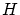- see Fig. 3.4) were installed along the periphery of a disc rotating at angular rate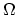.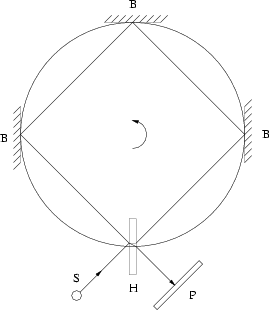A light beam was divided (by the plate) into two beams, and one beam traveled counterclockwise (in the direction of rotation) while the other traveled clockwise. An interference was observed at meeting of these beams. The fringe shift (as a result of the difference in times of propagation of light beams) had magnitude: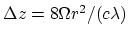. It is obvious that the non-inertial character of the system rotating atis of no concern: nobody saw a curved light beam in vacuum; light travels between two reflections rectilinearly. Nevertheless, we consider the following mental experiment: Imagine that the disc radius tends to infinity, but the value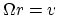remains constant. Then we have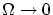. Therefore, the value of the acceleration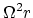tends to zero. Let us choose a radiussuch that the acceleration is much less than any pre-specified value (the existing experimental accuracy, for example). Nobody can distinguish this "near-inertial" system from a true inertial system. If the number of equidistant mirrors is also increased (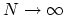), then the straight line (of light beams) between mirrors approaches the disc circle. As a result the fringe shift can be expressed as, where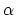is a constant for a given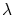andis the circumference. Because of the obvious symmetry of the experiment, the effect is additive in, and its value can be related to the unit length. A "cumulative" effect of acceleration can be made less than any pre-specified value for a given straightline region. Thus, we have for the magnitude of the fringe shift:(some variations inproduce appropriate variations in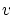, since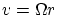is a finite value). Therefore, the time of signal propagation linearly depends on the velocity of the motion of the system, that is,.Next: Say a good word Up: Criticism of the relativistic experiments Previous: The Ritz hypothesis   Contents
Sergey N. Arteha